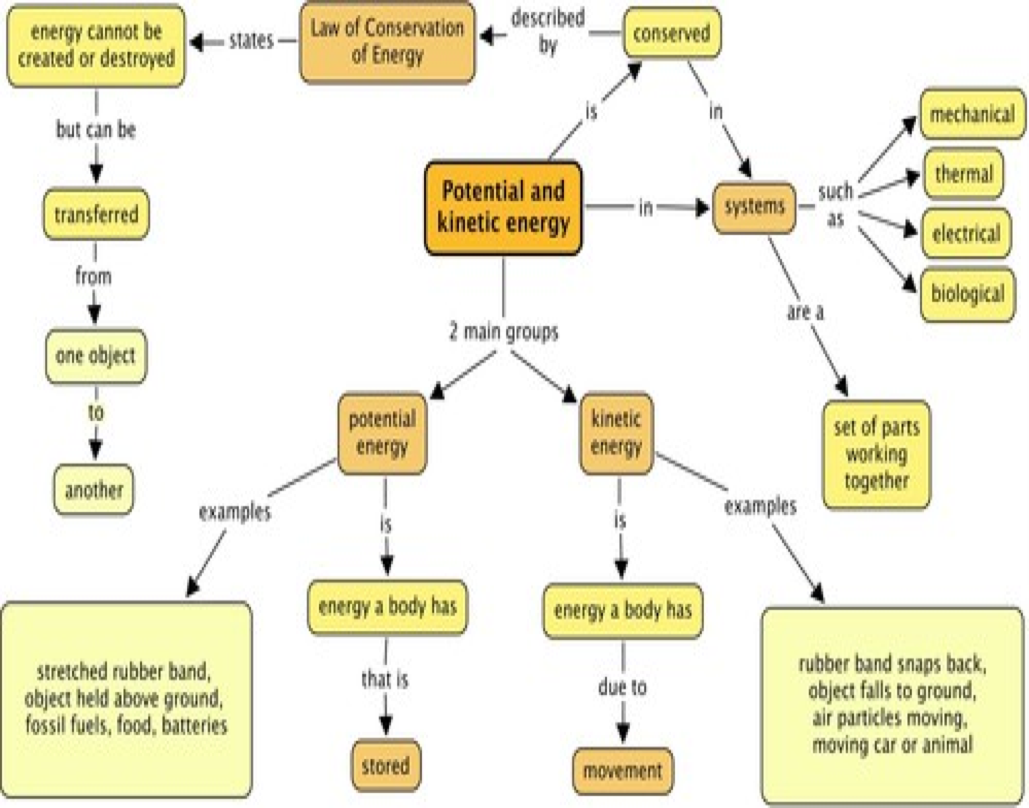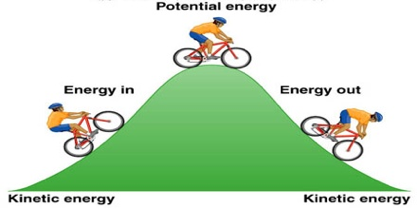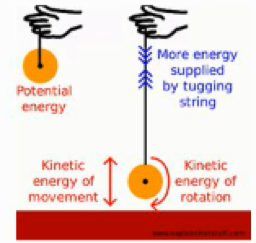# Potential and Kinetic Energy

### “All energy can be either in two forms, i.e. kinetic energy or potential energy”

All energy can be either in two forms, i.e. kinetic energy or potential energy. Energy can be transferred from potential energy to kinetic energy and kinetic energy to potential energy.

### Definition of Potential energy

• Potential energy is defined as the energy of the object when it is at rest and waiting to be released. The word ‘potential’ means the energy which has the ability to do something useful later on.

• The stored energy is generally called as potential energy.

• The potential energy PE of an object is equal to the mass m , g  gravitational acceleration of the earth and h  height above earth’s surface.#### Examples of potential energy are:

• A rock at the top of the hill
• Food for our bodies
• Fuel for our automobiles
• An arrow being stretched by a bow before it is released

### Potential Energy to Kinetic Energy Conversion

Potential Energy is converted into kinetic energy. Some examples are given below.A biker while going up on a hill has kinetic energy. When he reaches its maximum, he possesses potential energy when he is rest for a while. Again he gives out kinetic energy while moving down a hill. Similarly, yo-yo goes from potential energy to kinetic energy as it goes up and down.

### Relation between kinetic energy and potential energy

We know that potential energy is the energy that is stored within an object while kinetic energy is the energy that is in motion. The connection between the two is that potential energy transforms into kinetic energy. This can be seen in an example such as a book sitting on a counter. When the book is at rest, it has potential energy. But, say, when a person walks by and accidentally knocks the book off of the counter, the book has kinetic energy as it falls, because it is in motion and the potential energy has transformed into kinetic energy